# Electric and magnetic fields of a moving charge

milkism
Homework Statement:
Say two point charges q and −q moving with velocity v in opposite directions and
crossing each other at the origin at t = 0.
Relevant Equations:
Electric and magnetic field lines of moving charges.
Part a)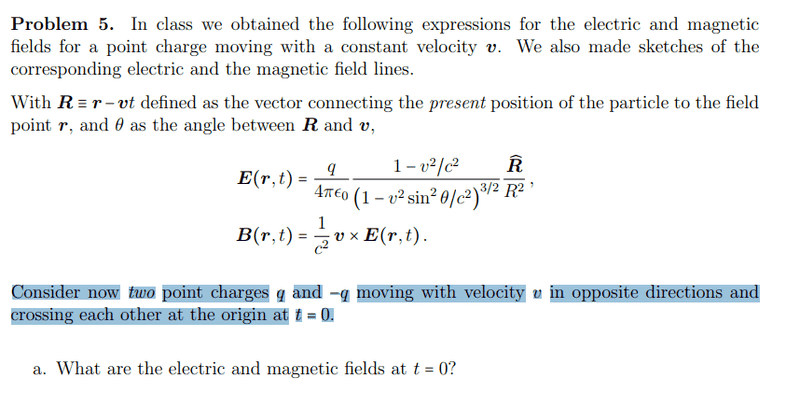My solution: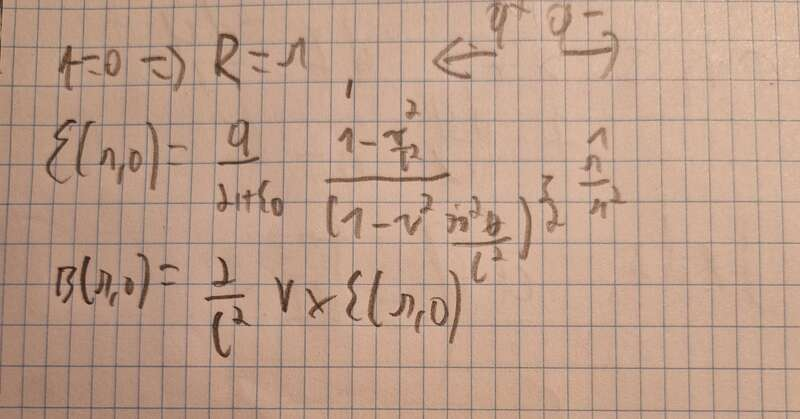Big R basically becomes r, and the electric and magnetic field lines are doubled because of superposition principle. Am I right?

Homework Helper
Gold Member
Homework Statement:: Say two point charges q and −q moving with velocity v in opposite directions and
crossing each other at the origin at t = 0.
Relevant Equations:: Electric and magnetic field lines of moving charges.

Part a)
View attachment 323356
My solution:
View attachment 323357

Big R basically becomes r, and the electric and magnetic field lines are doubled because of superposition principle. Am I right?
Not so fast. What are ##\mathbf{\hat{R}}_1## and ##\mathbf{\hat{R}}_2## for the two particles? Yes, there is superposition but you have to do it formally, not "basically."

Homework Helper
Gold Member
Big R basically becomes r, and the electric and magnetic field lines are doubled because of superposition principle. Am I right?

The two particles are oppositely charged. Would both the electric and magnetic fields double compared to the case of the single particle?

milkism
The two particles are oppositely charged. Would both the electric and magnetic fields double compared to the case of the single particle?
Yes since they're going opposite directions, if they were at same direction, it wouldn't.

milkism
Not so fast. What are ##\mathbf{\hat{R}}_1## and ##\mathbf{\hat{R}}_2## for the two particles? Yes, there is superposition but you have to do it formally, not "basically."
Let's say a point charge above where they cross, and let's say Q1 is above Q2, then R1=R1 and R2=R1+x, with x distance between Q1 and Q2?

milkism
Let's say a point charge above where they cross, and let's say Q1 is above Q2, then R1=R1 and R2=R1+x, with x distance between Q1 and Q2?
And also wouldn't the velocity signs change too?

Homework Helper
Gold Member
Consider the formulas you provided in the first post for the electric field ##\mathbf{E}## and ##\mathbf{B}## of a single point charge ##q## moving with velocity ##\mathbf{v}##.

Would the direction of ##\mathbf{E}## at the field point at time ##t = 0## change if the particle moved in the opposite direction (but kept the same charge ##q##)? Would the direction of ##\mathbf{B}## at the field point change at time ##t = 0## if the particle moved in the opposite direction (but kept the same charge ##q##)?

Would the direction of ##\mathbf{E}## at the field point change if the particle's charge was switched to ##-q## with no change in direction of velocity? Would the direction of ##\mathbf{B}## at the field point change if the particle's charge was switched to ##-q## with no change in direction of velocity?

milkism
Consider the formulas you provided in the first post for the electric field ##\mathbf{E}## and ##\mathbf{B}## of a single point charge ##q## moving with velocity ##\mathbf{v}##.

Would the direction of ##\mathbf{E}## at the field point at time ##t = 0## change if the particle moved in the opposite direction (but kept the same charge ##q##)? Would the direction of ##\mathbf{B}## at the field point change at time ##t = 0## if the particle moved in the opposite direction (but kept the same charge ##q##)?

Would the direction of ##\mathbf{E}## at the field point change if the particle's charge was switched to ##-q## with no change in direction of velocity? Would the direction of ##\mathbf{B}## at the field point change if the particle's charge was switched to ##-q## with no change in direction of velocity?
For first E no, first B yes, because of the cross product -v x E.
For second both yes.

Homework Helper
Gold Member
For first E no, first B yes, because of the cross product -v x E.
For second both yes.
Ok. So, if the direction of velocity is changed and the sign of the charge is also changed, what happens to the direction of E and the direction of B at the field point?

milkism
Ok. So, if the direction of velocity is changed and the sign of the charge is also changed, what happens to the direction of E and the direction of B at the field point?
E would change, B wouldn't?

Homework Helper
Gold Member
E would change, B wouldn't?
That's right.

milkism
That's right.
Resultant E will be zero then?

Homework Helper
Gold Member
Resultant E will be zero then?
Yes. Do you feel uncertain about making this conclusion?

milkism
Yes. Do you feel uncertain about making this conclusion?
No, but will R, or r be the same for both particles? With crossing each other do they mean that the two charges "touch" each other, or that they cross each other "parallelly"?

milkism
Or we can approximate by saying that the distance x between them is very small and we can ignore it.

Homework Helper
Gold Member
Or we can approximate by saying that the distance x between them is very small and we can ignore it.
yes

milkism
yes
So my initial answer for B-field was right?

Homework Helper
Gold Member
So my initial answer for B-field was right?
You stated that the B-field at the field point for the two charges at ##t = 0## would double compared to the single charge. That's right.

But it looks to me that your mathematical expression for ##\vec B## for the two particles that you wrote in post #1 is quadruple the field of the single charge.

My solution:
View attachment 323357
Your expression for E(r, 0) is twice that for a single charge. But you include an additional factor of 2 when writing B(r,0).

milkism
You stated that the B-field at the field point for the two charges at ##t = 0## would double compared to the single charge. That's right.

But it looks to me that your mathematical expression for ##\vec B## for the two particles that you wrote in post #1 is quadruple the field of the single charge.

Your expression for E(r, 0) is twice that for a single charge. But you include an additional factor of 2 when writing B(r,0).
Oh yes! Didn't see I meant to write double mathematically.

milkism
You stated that the B-field at the field point for the two charges at ##t = 0## would double compared to the single charge. That's right.

But it looks to me that your mathematical expression for ##\vec B## for the two particles that you wrote in post #1 is quadruple the field of the single charge.

Your expression for E(r, 0) is twice that for a single charge. But you include an additional factor of 2 when writing B(r,0).
Hey, don't know if I should make a new thread, but the next question is part b of the problem. It just asks to sketch the magnetic- and electric field lines of the moving charges before or after they cross each other. This is what I got: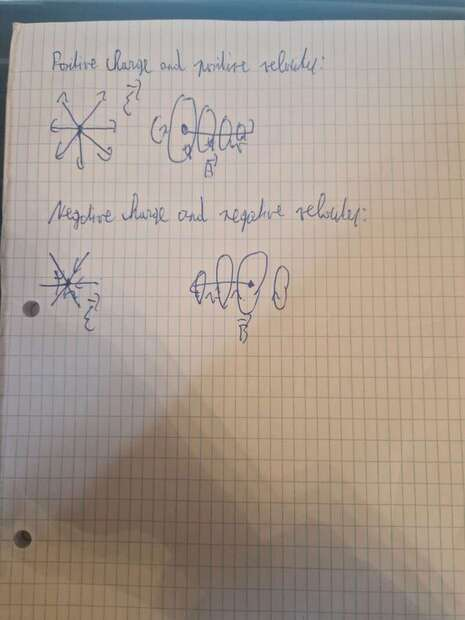For the positive charge, the electric field lines ofcourse "move away" from the charge, and the magnetic field lines make a circulation around the charge, for the positive charge in positive direction the magnetic field lines will first go out the page then go inside the page, because of the right-hand rule.
For negative charge and negative velocity, it would be just the other way right?

Homework Helper
Gold Member
Are they asking for a sketch of the net electric and magnetic fields due to both particles?

milkism
Are they asking for a sketch of the net electric and magnetic fields due to both particles?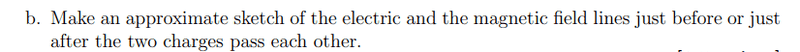I don't think so.

Homework Helper
Gold Member
milkism
yes
Sadly we may not, so my new equations became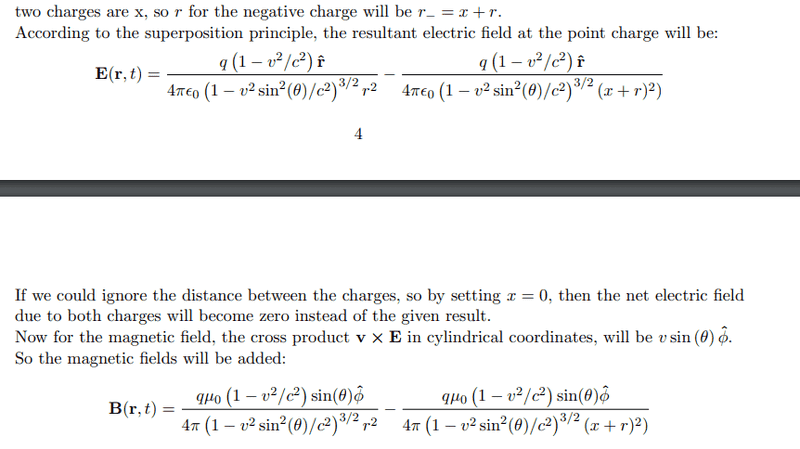For B(r,t) it should be + instead of -, my fault. Are these right?

milkism
Sadly we may not, so my new equations became View attachment 323644
For B(r,t) it should be + instead of -, my fault. Are these right?
Oh, yeah, also the angles would be different due to the distance x, right,?

milkism
It might be worth checking what is expected here.
It's net, this is what I got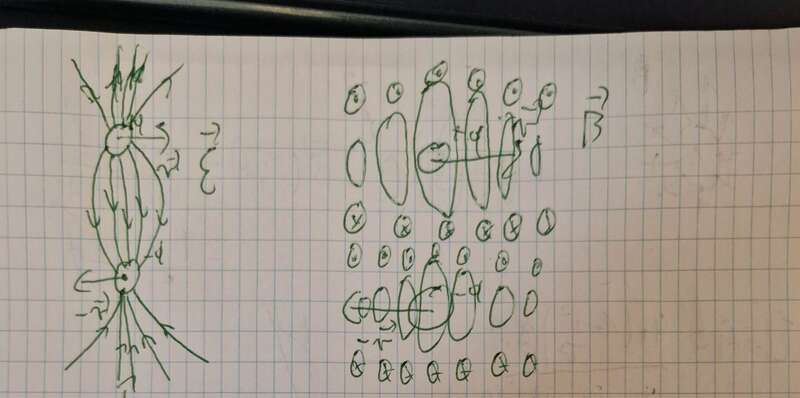milkism
Sadly we may not, so my new equations became View attachment 323644
For B(r,t) it should be + instead of -, my fault. Are these right?
Correction at cross product $$v\sin(\theta)E\mathbf{\hat{\phi}}$$

Homework Helper
Gold Member
Sadly we may not, so my new equations became View attachment 323644
Please type in your posts rather than post your discussion as images. Otherwise, it isn't easy to quote specific parts of your post. Posting images of diagrams is fine.

If the net electric field is not zero at the instant t = 0 when the two particles "cross", then I don't understand the setup of the problem. You say that Enet = 0 is not the "given result". If you can state the given result, it might help us interpret the setup.

You stated the following: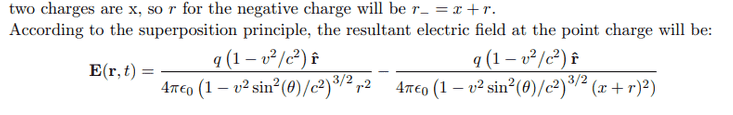What does it mean when you say "two charges are x"? Your formula for ##\mathbf{E}(\mathbf{r}, t)## must correspond to some configuration of the two charges. Can you post a carefully sketched diagram that shows the location of the two particles at the instant that corresponds to your formula? On the diagram, please indicate the location of the two particles and the location of the field point. Also, please label the quantities ##x##, ##r##, ##\hat {\mathbf{r}}##, and ##\theta##.

milkism
Please type in your posts rather than post your discussion as images. Otherwise, it isn't easy to quote specific parts of your post. Posting images of diagrams is fine.

If the net electric field is not zero at the instant t = 0 when the two particles "cross", then I don't understand the setup of the problem. You say that Enet = 0 is not the "given result". If you can state the given result, it might help us interpret the setup.

You stated the following:

What does it mean when you say "two charges are x"? Your formula for ##\mathbf{E}(\mathbf{r}, t)## must correspond to some configuration of the two charges. Can you post a carefully sketched diagram that shows the location of the two particles at the instant that corresponds to your formula? On the diagram, please indicate the location of the two particles and the location of the field point. Also, please label the quantities ##x##, ##r##, ##\hat {\mathbf{r}}##, and ##\theta##.
"The distance between the two charges are x" was the full sentence. The given result is just the expression I got for E(r,t).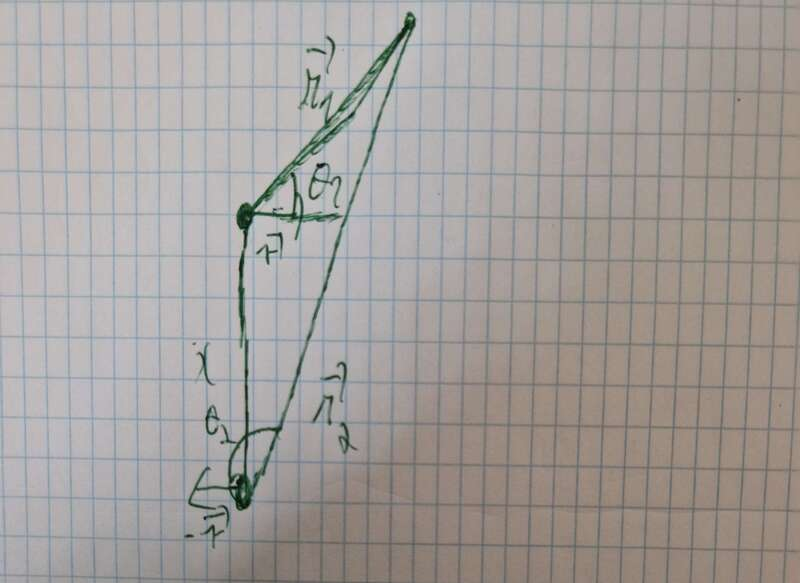When drawing the figure I just realized $$\mathbf{r_2}$$, which connects the negative charge to the field point is not $$\mathbf{r_1}+\mathbf{x}$$. That would have been the case if the field point were to be right above the charges, with both angles being zero. Finding an expression for $$\mathbf{r_2}$$ will be difficult, so I will just leave it as $$\mathbf{r_2}$$. So the electric-and magnetic field lines will look like:
$$\mathbf{E}(\mathbf{r},t) = \frac{q \left(1 - v^2 / c^2 \right) \mathbf{\hat{r_1}}}{4 \pi \epsilon_0 \left( 1 - v^2 \sin^2 (\theta_1 )/c ^2 \right)^{3/2}r_{1}^2} - \frac{q \left(1 - v^2 / c^2 \right) \mathbf{\hat{r_2}}}{4 \pi \epsilon_0 \left( 1 - v^2 \sin^2 (\theta_2 )/c ^2 \right)^{3/2}r_{2}^2}$$
$$\mathbf{B}(\mathbf{r},t) = \frac{q \mu_0 \left(1 - v^2 / c^2 \right) \sin(\theta_1) \mathbf{\hat{\phi}}}{4 \pi \left( 1 - v^2 \sin^2 (\theta_1 )/c ^2 \right)^{3/2}r_{1}^2} + \frac{q \mu_0 \left(1 - v^2 / c^2 \right) \sin(\theta_2) \mathbf{\hat{\phi}}}{4 \pi \left( 1 - v^2 \sin^2 (\theta_2 )/c ^2 \right)^{3/2}r_{2}^2}.$$
I guess.

Last edited:
milkism
Please type in your posts rather than post your discussion as images. Otherwise, it isn't easy to quote specific parts of your post. Posting images of diagrams is fine.

If the net electric field is not zero at the instant t = 0 when the two particles "cross", then I don't understand the setup of the problem. You say that Enet = 0 is not the "given result". If you can state the given result, it might help us interpret the setup.

You stated the following:

What does it mean when you say "two charges are x"? Your formula for ##\mathbf{E}(\mathbf{r}, t)## must correspond to some configuration of the two charges. Can you post a carefully sketched diagram that shows the location of the two particles at the instant that corresponds to your formula? On the diagram, please indicate the location of the two particles and the location of the field point. Also, please label the quantities ##x##, ##r##, ##\hat {\mathbf{r}}##, and ##\theta##.
Also I'm a bit confused with what they mean with "at origin" does that mean that the field point is right above the charges or?

Homework Helper
Gold Member
"The distance between the two charges are x" was the full sentence. The given result is just the expression I got for E(r,t).
View attachment 323650
When drawing the figure I just realized $$\mathbf{r_2}$$, which connects the negative charge to the field point is not $$\mathbf{r_1}+\mathbf{x}$$. That would have been the case if the field point were to be right above the charges, with both angles being zero. Finding an expression for $$\mathbf{r_2}$$ will be difficult, so I will just leave it as $$\mathbf{r_2}$$. So the electric-and magnetic field lines will look like:
$$\mathbf{E}(\mathbf{r},t) = \frac{q \left(1 - v^2 / c^2 \right) \mathbf{\hat{r_1}}}{4 \pi \epsilon_0 \left( 1 - v^2 \sin^2 (\theta_1 )/c ^2 \right)^{3/2}r_{1}^2} - \frac{q \left(1 - v^2 / c^2 \right) \mathbf{\hat{r_2}}}{4 \pi \epsilon_0 \left( 1 - v^2 \sin^2 (\theta_2 )/c ^2 \right)^{3/2}r_{2}^2}$$
$$\mathbf{B}(\mathbf{r},t) = \frac{q \mu_0 \left(1 - v^2 / c^2 \right) \sin(\theta_1) \mathbf{\hat{\phi}}}{4 \pi \left( 1 - v^2 \sin^2 (\theta_1 )/c ^2 \right)^{3/2}r_{1}^2} + \frac{q \mu_0 \left(1 - v^2 / c^2 \right) \sin(\theta_2) \mathbf{\hat{\phi}}}{4 \pi \left( 1 - v^2 \sin^2 (\theta_2 )/c ^2 \right)^{3/2}r_{2}^2}.$$
I guess.
As a vector equation, you could write ##\mathbf{r}_2 = \mathbf{x} + \mathbf{r}_1##, where ##\mathbf{x}## is the vector from ##q_2## to ##q_1##. So, $$r_2 = |\mathbf{x} + \mathbf{r}_1| = \sqrt{x^2 + r_1^2 + 2xr_1\sin\theta_1}$$ I'm not able to read your handwriting too well. In your diagram it looks like you are using the symbol ##\theta_2## for the angle of ##\mathbf{r}_1## and ##\theta_1## for the angle of ##\mathbf{r}_2##. It could just be my poor eyes not reading your subscripts on the angles very well .Otherwise, Your expression for the total E field looks good to me. The B field expression also looks good except I believe you dropped a factor of the speed ##v## in the numerators on the right side. Of course, I don't know if this form of writing the results is what is expected by the person who made up the problem.

•milkism
Homework Helper
Gold Member
Also I'm a bit confused with what they mean with "at origin" does that mean that the field point is right above the charges or?
Yes, I don't know how to interpret the statement of the problem. When I first read it, I thought the two charges are moving in opposite directions along the same line so that they meet at the origin at ##t = 0## and pass through each other. But I really don't know. I hesitate to try to go further with this problem until we can be clear on the interpretation of the problem statement. I don't want to lead you off in the wrong direction.

•milkism
Homework Helper
Gold Member
It's net, this is what I got View attachment 323645
OK. This looks about right for the net E field if the charges pass each other at some finite distance. For non-relativistic speeds, the electric field will be a dipole field as you've drawn. The E-field lines will be modified somewhat at relativistic speeds.

For the B field, you have indicated the fields of the two charges individually. It looks OK. It would not be easy to indicate the net B field from this perspective. Maybe you could sketch the net B field from the perspective of looking from the right so that the + charge is moving toward you and the - charge is moving away from you. So the plane of the page would be the plane passing through the charges and the plane oriented perpendicular to the direction of motion of the particles. The field lines of one of the charges alone would be circular. You could try to sketch the net B-field pattern.

•milkism
milkism
As a vector equation, you could write ##\mathbf{r}_2 = \mathbf{x} + \mathbf{r}_1##, where ##\mathbf{x}## is the vector from ##q_2## to ##q_1##. So, $$r_2 = |\mathbf{x} + \mathbf{r}_1| = \sqrt{x^2 + r_1^2 + 2xr_1\sin\theta_1}$$ I'm not able to read your handwriting too well. In your diagram it looks like you are using the symbol ##\theta_2## for the angle of ##\mathbf{r}_1## and ##\theta_1## for the angle of ##\mathbf{r}_2##. It could just be my poor eyes not reading your subscripts on the angles very well .Otherwise, Your expression for the total E field looks good to me. The B field expression also looks good except I believe you dropped a factor of the speed ##v## in the numerators on the right side. Of course, I don't know if this form of writing the results is what is expected by the person who made up the problem.
Thanks :D, yes theta_2 is for r_2 and theta_1 for r_1, and yep I forgot to add the velocity in the magnetic field lol. I have sent a message to the professor about the interpretation of "at the origin".